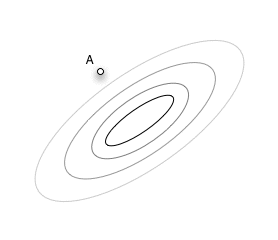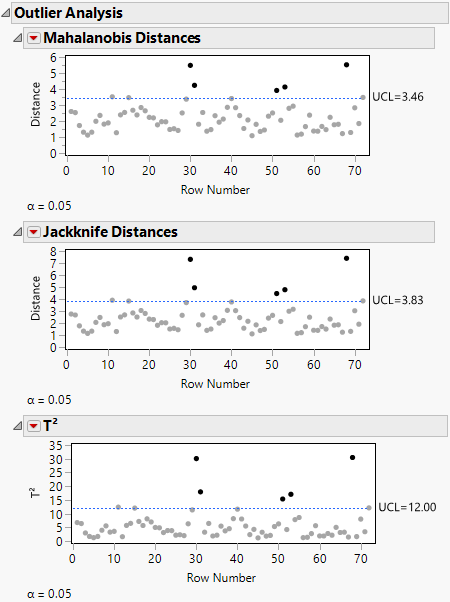Publication date: 08/13/2020

## Outlier Analysis

The Outlier Analysis menu contains options that each show or hide plots that measure distance in the multivariate sense, with respect to the correlation structure. For example, in Figure 3.5, Point A is an outlier because it is outside the correlation structure, even though it is not an outlier in any of the coordinate directions.

Figure 3.5 Example of an OutlierEach option in the Outlier Analysis menu shows or hides a plot of the corresponding distances. Testing is done at the alpha level that appears at the bottom of the plot. The following options are available:

Mahalanobis Distances

Shows or hides the Mahalanobis distance of each point from the multivariate mean (centroid). The standard Mahalanobis distance depends on estimates of the mean, standard deviation, and correlation for the data. The distance is plotted for each observation number. Extreme multivariate outliers can be identified by highlighting the points with the largest distance values. See Mahalanobis Distance Measures.

Jackknife Distances

Shows or hides distances that are calculated using a jackknife technique. The distance for each observation is calculated with estimates of the mean, standard deviation, and correlation matrix that do not include the observation itself. The jack-knifed distances are useful when there is an outlier. In this case, the Mahalanobis distance is distorted and tends to disguise the outlier or make other points look more outlying than they are. See Jackknife Distance Measures.

T2

Shows or hides distances that are the square of the Mahalanobis distance. This plot is preferred for multivariate control charts. The plot includes the value of the calculated T2 statistic, as well as its upper control limit. Values that fall outside this limit might be outliers. See T2 Distance Measures.

Figure 3.6 Outlier Analysis Plots### Saving Distances and Values

You can save any of the distances to the data table by selecting the Save option from the red triangle menu for the plot.

Note: There is no formula saved with the jackknife distance column. This means that the distance is not recomputed if you modify the data table. If you add or delete columns, or change values in the data table, select Analyze > Multivariate Methods > Multivariate again to compute new jackknife distances.

In addition to saving the distance values for each row, a column property is created that holds the upper control limit (UCL) value for the Outlier Analysis type specified.